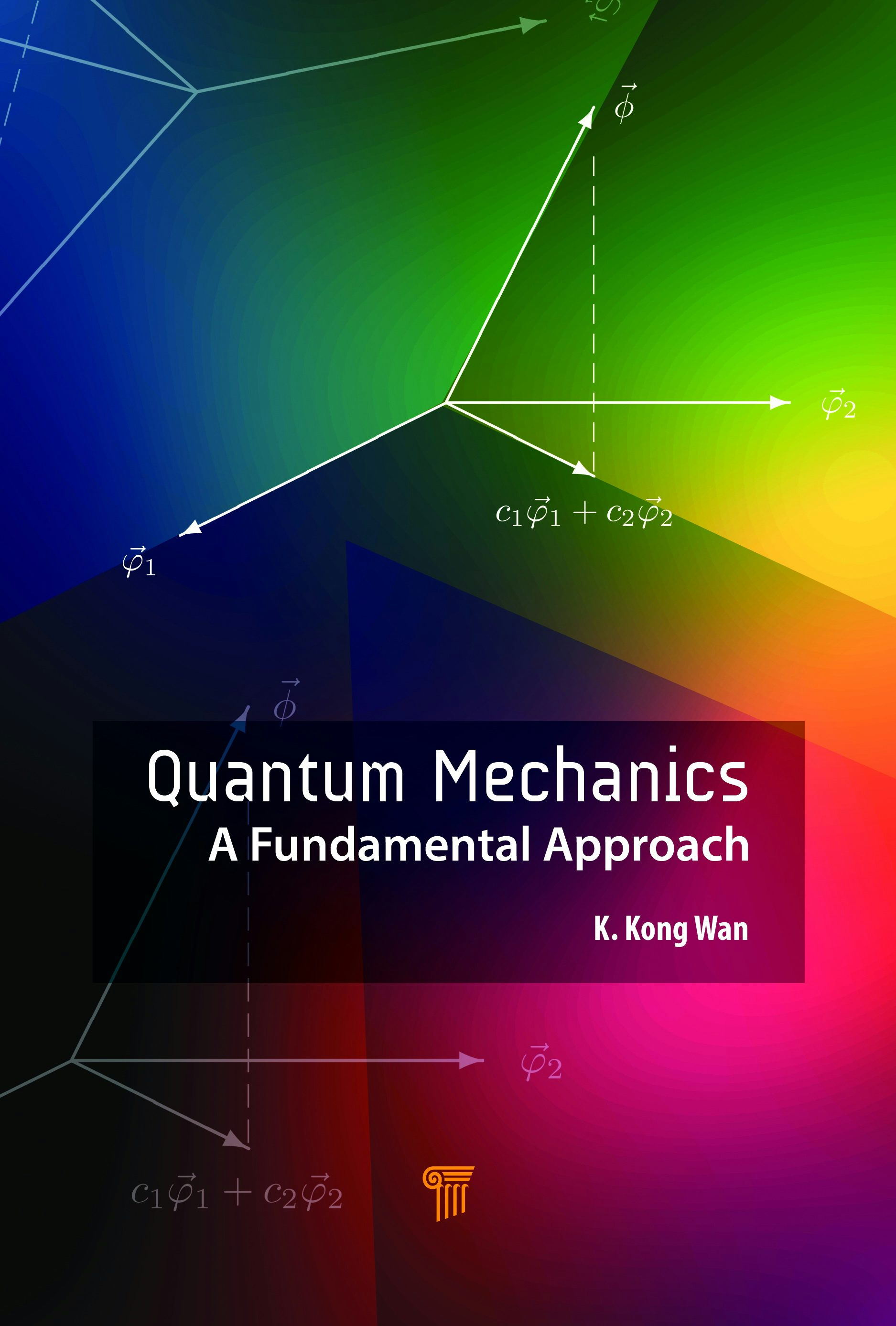# Quantum Mechanics

## A Fundamental Approach

by Kong Wan

350 pages

• Hardcover
• ISBN: 9789814774659
• Published: June 2019

• eBook - PDF
• ISBN: 9780203702413

Retailers:

The mathematical formalism of quantum theory in terms of vectors and operators in infinite-dimensional complex vector spaces is very abstract. The definitions of many mathematical quantities used do not seem to have an intuitive meaning. This makes it difficult to appreciate the mathematical formalism and hampers the understanding of quantum mechanics. This book provides intuition and motivation to the mathematics of quantum theory, introducing the mathematics in its simplest and familiar form, for instance, with three-dimensional vectors and operators, which can be readily understood. Feeling confident about and comfortable with the mathematics used helps readers appreciate and understand the concepts and formalism of quantum mechanics.

Quantum mechanics is presented in six groups of postulates. A chapter is devoted to each group of postulates with a detailed discussion. Systems with superselection rules, and some conceptual issues such as quantum paradoxes and measurement, are also discussed. The book concludes with several illustrative applications, which include harmonic and isotropic oscillators, charged particle in external magnetic fields and the Aharonov–Bohm effect.